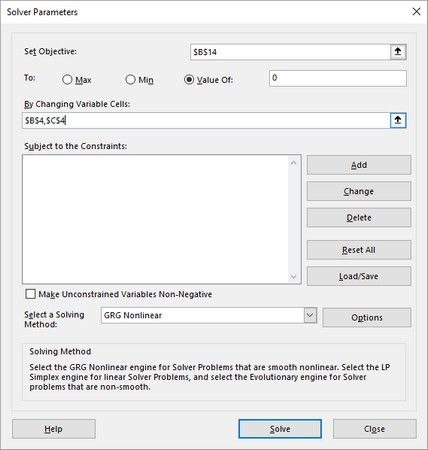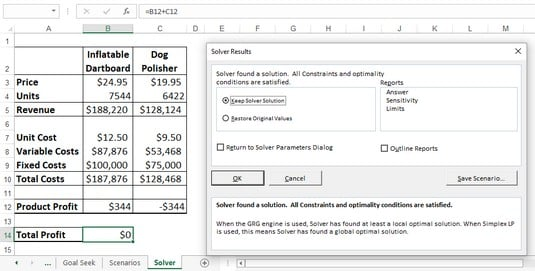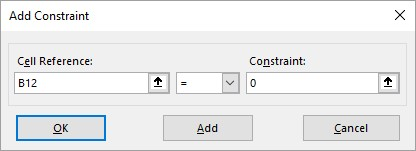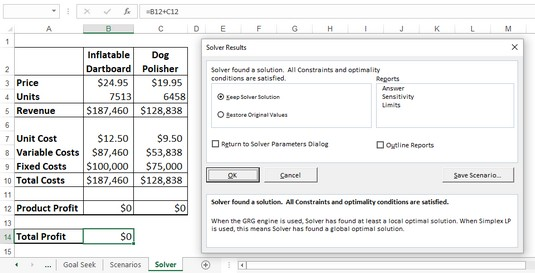##### Excel Data Analysis For DummiesYou set up your Excel Solver model by using the Solver Parameters dialog box. You use the Set Objective box to specify the objective cell, and you use the To group to tell Excel Solver what you want from the objective cell: the maximum possible value; the minimum possible value; or a specific value. Finally, you use the By Changing Variable Cells box to specify the cells that Solver can use to plug in values to optimize the result.

## Optimizing Excel Solver results

When Solver finds a solution, you can choose either Keep Solver Solution or Restore Original Values. If you choose Keep Solver Solution, Excel permanently changes the worksheet. You cannot undo the changes.

1. Choose Data → Solver.

Excel opens the Solver Parameters dialog box.

2. In the Set Objective box, enter the address of your model’s objective cell.

Note that if you click the cell to enter it, Solver automatically enters an absolute cell address (for example, `\$B\$14` instead of `B14`). Solver works fine either way.

3. In the To group, select an option:
• Max: Returns the maximum possible value.
• Min: Returns the minimum possible value.
• Value Of: Enter a number to set the objective cell to that number.

For the example model, Value Of has been selected and 0 entered in the text box.

4. In the By Changing Variable Cells box, enter the addresses of the cells you want Solver to change while it looks for a solution.

In the example, the changing cells are B4 and C4. The following image shows the completed Solver Parameters dialog box.The completed Solver Parameters dialog box.
5. Click Solve.

Solver gets down to business. As Solver works on the problem, you might see the Show Trial Solution dialog boxes show up one or more times.

6. In any Show Trial Solution dialog box that appears, click Continue to move things along.

When the optimization is complete, Excel displays the Solver Results dialog box.The Solver Results dialog box and the solution to the break-even problem.
7. Select the Keep Solver Solution option.

If you don't want to accept the result, select the Restore Original Values option instead.

8. Click OK.

You can ask Solver to display one or more reports that give you extra information about the results. In the Solver Results dialog box, use the Reports list to select each report you want to view:

• Answer: Displays information about the model’s objective cell, variable cells, and constraints. For the objective cell and variable cells, Solver shows the original and final values.
• Sensitivity: Attempts to show how sensitive a solution is to changes in the model’s formulas. The layout of the Sensitivity report depends on the type of model you’re using.
• Limits: Displays the objective cell and its value, as well as the variable cells and their addresses, names, and values.

Excel Solver can use one of several solving methods. In the Solver Parameters dialog box, use the Select a Solving Method list to select one of the following:

• Simplex LP: Use if your worksheet model is linear. In the simplest possible terms, a linear model is one in which the variables are not raised to any powers and none of the so-called transcendent functions — such as SIN and COS — are used.
• GRG Nonlinear: Use if your worksheet model is nonlinear and smooth. In general terms, a smooth model is one in which a graph of the equation used doesn’t show sharp edges or breaks.
• Evolutionary: Use if your worksheet model is nonlinear and nonsmooth.
Do you have to worry about any of this? Almost certainly not. Excel Solver defaults to using GRG Nonlinear, and that should work for almost anything you do with Solver.

## Adding constraints to Excel Solver

The real world puts restrictions and conditions on formulas. A factory might have a maximum capacity of 10,000 units a day, the number of employees in a company can’t be a negative number, and your advertising costs might be restricted to 10 percent of total expenses.

Similarly, suppose that you’re running a break-even analysis on two products. If you run the optimization without any restrictions, Solver might reach a total profit of 0 by setting one product at a slight loss and the other at a slight profit, where the loss and profit cancel each other out. In fact, if you take a close look at the previous image, this is exactly what Solver did. To get a true break-even solution, you might prefer to see both product profit values as 0.

Such restrictions and conditions are examples of what Solver calls constraints. Adding constraints tells Solver to find a solution so that these conditions are not violated.

Here’s how to run Solver with constraints added to the optimization:

1. Choose Data → Solver.

Excel opens the Solver Parameters dialog box.

2. Use the Set Objective box, the To group, and the By Changing Variable Cells box to set up Solver as described above.

Excel displays the Add Constraint dialog box.

4. In the Cell Reference box, enter the address of the cell you want to constrain.

You can type the address or select the cell on the worksheet.

5. In the drop-down list, select the operator you want to use.

Most of the time, you use a comparison operator, such as equal to (`=`) or greater than (`>`). Use the `int` (integer) operator when you need a constraint, such as total employees, to be an integer value instead of a real number (that is, a number with a decimal component; you can't have 10.5 employees!). Use the `bin` (binary) operator when you have a constraint that must be either `TRUE` or `FALSE` (or 1 or 0).

6. If you chose a comparison operator in Step 5, in the Constraint box, enter the value by which you want to restrict the cell.

This image shows an example of a completed Add Constraint dialog box. In the example model, this constraint tells Solver to find a solution such that the product profit of the Inflatable Dartboard (cell B12) is equal to 0.The completed Add Constraint dialog box.
7. To specify more constraints, click Add and repeat Steps 4 through 6, as needed.

For the example, you add a constraint that asks for the Dog Polisher product profit (cell C12) to be 0.

8. Click OK.

Excel returns to the Solver Parameters dialog box and displays your constraints in the Subject to the Constraints list box.

9. Click Solve.
10. In any Show Trial Solution dialog box that appears, click Continue to move things along.

The image below shows the example break-even solution with the constraints added. Notice that not only is the Total Profit cell (B14) set to 0, but so are the two Product Profit cells (B12 And C12).The Solver Results dialog box and the final solution to the break-even problem.
11. Select the Keep Solver Solution option.

If you don’t want to accept the result, select the Restore Original Values option instead.

12. Click OK.

You can add a maximum of 100 constraints. Also, if you need to make a change to a constraint before you begin solving, select the constraint in the Subject to the Constraints list box, click Change, and then make your adjustments in the Change Constraint dialog box that appears. If you want to delete a constraint that you no longer need, select the constraint and then click Delete.

## Save an Excel Solver solution as a scenario

Whenever you have a spreadsheet model that uses a coherent set of input values — known as changing cells — you have what Excel calls a scenario. With Solver, these changing cells are its variable cells, so a Solver solution amounts to a kind of scenario in Excel. However, Solver does not give you an easy way to save and rerun a particular solution. To work around this problem, you can save a solution as a scenario that you can then later recall using Excel’s Scenario Manager feature.

Follow these steps to save a Solver solution as a scenario:

1. Choose Data → Solver.

Excel opens the Solver Parameters dialog box.

2. Use the Set Objective box, the To group, the By Changing Variable Cells box, and the Subject to the Constraints list to set up Solver as described above.
3. Click Solve.
4. Anytime the Show Trial Solution dialog box appears, choose Continue.

When the optimization is complete, Excel displays the Solver Results dialog box.

5. Click Save Scenario.

Excel displays the Save Scenario dialog box.

6. In the Scenario Name dialog box, type a name for the scenario and then click OK.

Excel returns you to the Solver Results dialog box.

7. Select the Keep Solver Solution option.

If you don’t want to accept the result, select the Restore Original Values option instead.

8. Click OK.